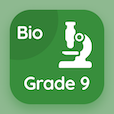Engineering Online Courses

Engineering Mathematics MCQs

Engineering Mathematics Practice Problems with Answers Multiple Choice Questions (MCQ), Engineering Mathematics quiz answers PDF to study engineering mathematics online course for engineering mathematics classes. Linear Algebra Multiple Choice Questions and Answers (MCQs), Engineering Mathematics quiz questions for pre employment screening tests. "Engineering Mathematics Practice Problems with Answers" PDF Book: differential equations final exam questions with solutions, advanced maths quiz questions with answers, differential equations questions with solutions, differential equations final exam with solutions test prep for online associate degree in engineering.

"Matrix which is a square matrix whose transpose equals its negative is called" MCQ PDF: engineering mathematics practice problems with answers with choices symmetric matrix, skew symmetric matrix, augmented matrix, and triangular matrix for pre employment screening tests. Study engineering mathematics practice problems with answers quiz questions for merit scholarship test and certificate programs for online college admission.

## MCQs on Engineering Mathematics Practice Problems with Answers Quiz

MCQ: Matrix which is a square matrix whose transpose equals its negative is called

symmetric matrix
skew symmetric matrix
augmented matrix
triangular matrix

MCQ: Eigenvalue of a square matrix A are the roots of

differential equation
characteristic equation
integral equation
logical equation

MCQ: A matrix which is formed by turning all the rows of a given matrix into columns and vice-versa is called

skew matrix
transpose matrix
augmented matrix
identity matrix

MCQ: Each n x n matrix must have at least

one eigenvalue
two eigenvalues
three eigenvalues
four Eigen values

MCQ: Eigenvalues of symmetric matrix are

imaginary
real
string
characters

### More Topics from Engineering Mathematics Course